﻿ Planck Gravitation TheoryPublications are Open
Access in this journal
Article Versions
Export Article
• Normal Style
• MLA Style
• APA Style
• Chicago Style
Original Article
Open Access Peer-reviewed

### Planck Gravitation Theory

XiaoLin Li
International Journal of Physics. 2019, 7(4), 118-125. DOI: 10.12691/ijp-7-4-2
Received October 02, 2019; Revised November 10, 2019; Accepted November 26, 2019

### Abstract

A new gravitation theory, Planck gravitation theory. Gravitation is a quantum force in 4-dimensional space. Gravitation is not actually related to the mass of particles. Every quantum particle produces the same strength of gravitation, regardless of the type of particle. The origin of gravitation is quantum, and gravitation is already a quantum force. In 4-dimensional space, gravitation is inversely proportional to the cubic of distance, not square of distance. The strength of gravitation is entirely determined by the Planck Length. The Planck Length is the identification constant of gravitation. The author's earlier projection gravitation theory is only a derivation of the Planck gravitation theory. Planck gravitation is separated into two different patterns in 3-dimensional space. For particles with rest mass, Planck gravitation translates into projection gravitation, which is inversely proportional to the square of distance. The Newton gravitation is a low-speed approximation of the projection gravitation. For particles with zero rest mass, gravitation is inversely proportional to the cubic of distance. For example, the gravitation of photons is inversely proportional to the cubic of distance. Every quantum particle is an empty hole in space, and the radius of empty hole is Planck Length. This brings the effect of the quantization of space-time. Planck gravitation theory can solve the problem of ultraviolet divergence in quantum field theory without the need for renormalization. If the Planck gravitation theory is true, human need to rethink the gravitation, and need to rethink the way of gravitation quantization. The author finally discusses the projection action, which is the key to human understanding of the truth about gravitation.

### 1. Introduction

In the author's previous paper 1, author has proposed a projection gravitation theory. Gravitation is a projection force from 5-dimensional space-time into 4-dimensional space-time. From the projection gravitation theory, we can get similar results to general relativity. From the projection gravitation theory, we can explain these phenomena, such as the deflection of light by the sun, the precession of mercury, the red shift of light by the sun, the radar echo delay.

From the projection gravitation theory, we can derive out a very simple model of black hole. From the model of black hole, we can easily derive out the black hole entropy formula 2, 3. And we can easily derive out the Verlinde’s entropy gravity proposal formula and the Unruh formula. We can prove that the Verlinde’s entropy gravity proposal formula and the Unruh formula are actually equivalent. The Verlinde’s entropy gravity proposal formula is only a derivation of black hole entropy. It is not an independent gravitation theory.

From the projection gravitation theory, we can derive a lot of meaningful results. The projection gravitation theory is an independent and rational theory of gravity. More importantly, the principle of projection gravity theory is fully consistent with quantum mechanics, and the derivation of black hole entropy is based on quantum mechanics. There is no contradiction between projection gravitation theory and quantum mechanics. The projection gravitation theory is a self-contained theory of quantum gravity.

However, in projection gravitation theory, there are two big problems that have not been solved.

(1). Since gravitation is a projection force from 4-dimensional space into 3-dimensional space, so what properties does gravitation have in 4-dimensional space?

(2). In the projection gravitation theory, the author thinks that only rest mass can produce gravitation, and particles without rest mass do not produce gravitation. Photons do not produce gravitation, not a source of gravity. Is this conclusion correct? What is the gravity produced by photons?

In the study of these two problems, the authors propose a more basic theory of gravitation. The authors find that gravitation exists in 4-dimensional space. Gravitation is purely a quantum effect. Gravitation itself actually comes from quantum. The projection gravitation theory is also the result of this new gravitation theory. This new gravitation theory is the real basic theory of gravitation. And this new gravitation theory provides a clear answer to these two questions.

### 2. Planck Gravitation Theory

In the projection gravitation theory 1, it contains the following three basic concepts.

(1). Quantum waves exist in 4-dimensional space. The group velocity of quantum waves is equal to the phase velocity, the velocity value is constant, and the velocity value is the speed of light. To this property, author named it as particle-wave symmetry. In 4-dimensional space, all particle waves have same value of speed. The value is the speed of light. This phenomenon is called speed quantization. The speed of light in 3-dimensional space is just a special example of speed quantization.

(2). Projecting particle waves in 4-dimensional space into 3-dimensional space, this brings Lorentz symmetry. The physical essence of Lorentz symmetry is speed quantization. The speed of light is a quantization speed. The momentum component of a particle in the 4th space dimension is projected into 3-dimensional space, which is the rest mass of the particle in 3-dimensional space. The Compton wavelength of the particle is the de Broglie wavelength of the particle in the 4th space dimension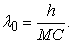M is the rest mass of a particle in 3-dimensional space.

(3). The gravitation in 3-dimensional space is a projection force. Gravitation is a projection force from 5-dimensional space-time into 4-dimensional space-time.

In projection gravitation theory, the rest mass of gravitational source is M. so the gravitational source has a property called radius of black hole. The radius is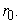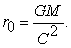(2.1)

There exists another particle B with rest mass of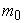is affected by the gravitation produced by M. The gravitation energy of B is (2.2).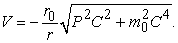(2.2)

The energy of the particles is (2.3).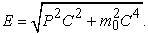(2.3)

So, particle B moves in the gravitation field, its total energy is (2.4).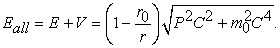(2.4)

The radius of black hole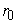characterizes the gravitation force.is actually a projection factor that characterizes the strength of the projection force. The larger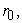the stronger the gravitation.is proportional to the rest mass of the gravitational source.

We change the form of (2.4), so get (2.5).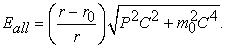(2.5)

Formula (2.5) is a form of energy-momentum expression of Lorentz symmetry. In projection gravitation theory, the Lorentz symmetry also is a projection effect. We change formula (2.3) to (2.6).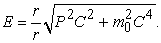(2.6)

This formula better reflects the essential nature of Lorentz symmetry. Lorentz symmetry is projection symmetry. Space is symmetrical, so projection is symmetrical. The projection factor is r/r=1. The projection factor is 1, this situation reflects the space symmetry.

Formula (2.5) can be seen as breaking to space symmetry.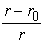can be seen as breaking to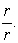The gravitational source has the property of radius of black hole. The black hole is actually an empty hole. The empty hole leads to a loss of space. The loss of space leads to the breaking to space symmetry. So the projection gravitation is actually a breaking effect of space symmetry. Space symmetry breaking leads to the breaking of Lorentz symmetry. So the projection gravitation is actually a breaking effect of Lorentz symmetry. However, this Lorentz symmetry breaking effect is very special. It is not changing the speed of light, but changing the projection. The essence of Lorentz symmetry is a projection from 4-dimensional space into 3-dimensional space. Space symmetry is broken, resulting in breaking of projection, and resulting in Lorentz symmetry breaking. This breaking leads to the gravitational effect. This is the physical essence of projection gravitation.

Readers who cannot understand the above explanation, please read the author's previous paper to understand the projection gravitation theory 1. Only after understanding the previous theory can you understand the following.

Please look closely at the projection factor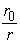in formula (2.2). Take (2.1) into (2.2), get (2.7).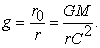(2.7)

The Compton wavelength formula for particles is (2.8).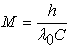(2.8)

Take (2.8) into (2.7), get (2.9).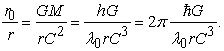(2.9)

Please note that the Planck Length appears here. There is a close relationship between projection gravitation and Planck Length.

Let's mark the Planck Length with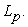We can simplify (2.9) and switch to (2.10).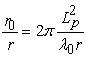(2.10)

This is the gravitation formula in 3-dimensional space.

In 4-dimensional space, the projection no longer exists. It is thought that the gravitational potential energy in 3-dimensional space is inversely proportional to r, then the gravitational potential energy in 4-dimensional space should be inversely proportional to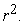Obviously, from (2.10), we can get a result. In 4-dimensional space, the force factor of gravitation should be expressed as (2.11).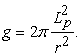(2.11)

Please note that r is the 4-dimensional distance between the particle and the gravitational source in formula (2.11).

Replacewith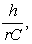so change (2.7) to (2.11). The momentum component of a particle in the 4th space dimension is projected into 3-dimensional space, which is the rest mass of the particle in 3-dimensional space. In 4-dimensional space, the projection no longer exists. Obviously,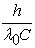should be replaced with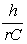in 4-dimensional space. So get (2.11).

Gravitation is a force in 4-dimensional space. There's a huge difference between gravitation and electromagnetic force. Electromagnetic force is a force in 3-dimensional space. But gravitation is a force in 4-dimensional space. The gravitation equation of particles is (2.12) in 4-dimensional space.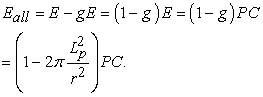(2.12)

In (2.12), r is a 4-dimensional distance, and momentum P is a 4-dimensional momentum.

Please note. Planck Length is a very special physical quantity.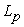is determined by three physical constants,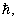G, and C. Three constants are Lorentz invariant. So, Planck Length is also a Lorentz invariant. So, gravitation has Lorentz invariance.

(2.12) and (2.4) are similar. The difference is (2.12) in 4-dimensional space and (2.4) in 3-dimensional space. So, we can understand (2.12) from this perspective. In 4-dimensional space, gravitation is a breaking effect of space symmetry. The reason for the breaking of space symmetry is the existence of particles. Each particle has an intrinsic property of Planck-Length. In 4-dimensional space, each particle is a black hole. In 4-dimensional space, the radius of the black hole of particles is same, the radius is just the Planck Length. Each particle has the same radius of the black hole, which is exactly the Planck Length. As long as particles exist, they can cause breaking to space symmetry. The breaking of space symmetry leads to the breaking of the symmetry of the wave function, so the gravitational effect occurs. The physical origin of the gravitational effect comes entirely from quantum mechanics. It is the quantization of particles that leads to gravitational effects. The physical nature of Planck Length is actually the intensity of the gravitational force. Planck Length is actually a special characteristic of the gravitation force.

Because the total energy of particles cannot be negative. Therefore, as can be seen from (2.12), there is a minimum distance between the particle and the gravitational center. This minimum distance can be identified by the Planck Length. Physical images, like space appear a hole. Particles will never enter the inner region of the hole. So, a black hole is actually an empty hole. A black hole does not inhale everything, but an infinite attraction to particles, but particles never enter the inner region of the hole. This is similar to projection gravitation.

As can be seen from (2.12), there is no correlation between gravitation and rest mass actually in 4-dimensional space. The gravitational factor g of any particle is identified by the Planck Length. And the strength of gravitation of any particle is same. Planck Length is the intensity of the gravitation, and Planck Length is a quantum value. So, gravitation is actually a quantum force. So, we need to rethink the problem of gravitation quantization.

There is a projection action from 4-dimensional space to the 3-dimensional space. The nature of the projection has not yet been revealed, and this is the next big problem. This projection action results in the presence of the rest mass of particles in 3-dimensional space. The physical origin of the rest mass is actually derived from this projection. The rest mass M is represented by (2.8). This projection action causes the distance r in the 4th space dimension to be fixed. This fixed distance is the Compton wavelength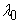in 3-dimensional space. It is because of this projection action that the gravitational factor (2.11) in 4-dimensional space is transformed into the gravitational factor (2.7) in 3-dimensional space. Therefore, the projection gravitation theory proposed earlier by the author is actually a derivation result of this new gravitation theory. This new gravitation theory is the basic theory.

This new gravitation theory is characterized entirely by Planck Length. Planck first proposed the concept of Planck Length. So, in honor of Planck, the author named the theory by Planck gravitation Theory. The physical nature of Planck Length is truly revealed in Planck gravitation theory.

It can be seen that gravitation is originally a quantum force in 4-dimensional space. It is because of the projection that the Planck constant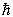in the gravitational factor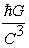is replaced by the rest mass of particle in 3-dimensional space. This hides the quantum origin of gravitation. This misleads humans so much that they believe that there is no relationship between gravitation and quantum mechanics. This has led to humans unable to understand the true origin of gravitation. The true origin of gravitation actually comes from quantum mechanics.

In 4-dimensional space, gravitation is not related to rest mass, and the gravitational strength of each particle is same. Gravitational strength is inversely proportional to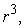notIn 3-dimensional space, gravitation is inversely proportional to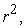which is actually a projection effect, not the real situation.

For gravitation in 3-dimensional space, the black hole radius of particles is (2.1). So, when a particle has a rest mass less than Planck Mass, its black hole radius is smaller than Planck Length. This seems to contradict Planck gravitation theory. But, in fact, this is also an illusion created by projection. In 3-dimensional space, the black hole radius of particles is (2.1). If the rest mass of the particle is less than Planck Mass, and the rest mass of the particle depends on the Compton wavelength of the particle. So, its Compton wavelength must be larger than Planck Length. Because Compton wavelengths are related to projection. Compton wavelength is larger than Planck Length, so the distance between particles is still greater than Planck Length in 4-dimensional space. As seen in 3-dimensional space, the distance between particles appears to be smaller than Planck Length. But this is only a projection illusion. In 4-dimensional space, the distance between particles is still greater than Planck Length. This problem, in fact, also reflects that gravitation actually has a certain degree of distortion in 3-dimensional space. Some of the results derived by gravitation in 3-dimensional space may not be exactly the truth. Only by calculating in 4-dimensional space can the results be completely true.

From (2.12), we can see why gravitation is a universal force. On the one hand, from the perspective of forced party to look at, gravitation is a breaking effect of space symmetry. Any particle, as long as it exists in space, will inevitably be affected by this kind of breaking effect, will inevitably be affected by the force of gravitation. On the other hand, from the perspective of the gravitational source to look at, gravitation is an accompanying result of particle quantization. Gravitation is determined only by the three constants of theG and C. Gravitation is actually a quantum force. Any quantum particle is a gravitational source, and any quantum particle has a gravitational effect on other particles. From Planck gravitation theory, it is more easily to see why gravitation is a universal force.

### 3. Gravitation of Photon

In the projection gravitation theory proposed earlier by the author, it is believed that only the rest mass has a projection effect, only the rest mass can produce gravitation, and the particles with zero rest mass do not produce gravitation. This is the deficiency of projection gravitation theory. Because projection gravitation theory is only an inference of Planck gravitation theory, not the whole of gravitation.

Look at this problem from Planck gravitation. Photons do not have momentum in the 4th space dimension, so photons do not have rest mass. The momentum of photons is fully projected into 3-dimensional space. Therefore, photons do not have Planck wavelength. So, Planck gravitation is all projected into 3-dimensional space without gravitational distortion. So, in 3-dimensional space, the gravitation of photons is Planck gravitation. The gravitational factor produced by photons in 3-dimensional space is (2.11), which is below (3.1).

Please note, in (3.1), the r is distance in 3-dimensional space, not in 4-dimensional space. (3.1) is a formula in 3-dimensional space. The gravitation produced by photons has nothing to do with the energy of photons. All photons produce the same strength of gravitation. This is very different from the General Relativity. This is also very different from Newton gravitation.

Other particles move in the photon gravitational field, and the total energy of the particles is (3.2).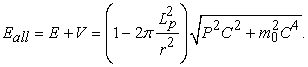(3.2)

Because the gravitational potential energy produced by photons is inversely proportional to thenot inversely proportional to the r. So, at the macro scale, the gravitation produced by photons is very weak. Compared with electromagnetic forces, it is extremely small.

However, it is precisely because the gravitational potential energy produced by photons is inversely proportional to theIn the microscale range, the strength gap of the force will be significantly reduced compared with the electromagnetic force. The smaller the distance r, the greater the gravitation force. As the Planck Length scale approaches, photons produce more gravitation forces than electromagnetic forces. This is a very shocking result. The gravitation produced by photons is weak at macro level, but powerful at the micro level.

In 3-dimensional space, other particles with zero rest mass produce exactly the same gravitation as photons.

The equation of gravitation produced by photons is derived out. This is one of the great achievements of Planck gravitation theory. The nature of the gravitation produced by photons has always been a question of no precise answer. Projection gravitation theory also does not give an answer to the gravitation of photons, which is a deficiency of projection gravitation. Planck gravitation theory just makes up for this. Projection gravitation is only an inference of Planck gravitation, not the whole of gravitation. It is Planck gravitation theory that can reveal the whole truth about gravitation.

Please note. Because of projection, Planck gravitation is separated into two different gravitation patterns in 3-dimensional space. For particles with rest mass, Planck gravitation translates into projection gravitation, which is inversely proportional toFor particles with zero rest mass, there is no change in Planck gravitation, and gravitation is inversely proportional to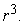This is an amazing property of Planck gravitation. This particular property of gravitation was revealed for the first time.

### 4. Ultraviolet Divergence in QFT

Because there can be no particle with a negative total energy, Planck gravitation theory has a surprising property. It is limited by Planck gravitation that the distance between the two particles can only be as long as Planck Length, not less than Planck Length. In 3-dimensinal space, photons are also subject to this limitation and have this particular property. The same is true for other particles with zero rest mass. This can be seen from (3.2).

In quantum field theory, it is precisely because the distance between particles is too small, can be infinitely small, can be close to 0, it brings the infinite increase of momentum, it brings the problem of ultraviolet divergence. Although the renormalization solves this problem, but after all, it is an artificial means, not from the basic principles to solve the problem.

Planck gravitation theory, however, can bring a minimum distance limit. The distance between the two particles is only as long as Planck Length. Therefore, the momentum of the particle must have a maximum value. The problem of ultraviolet divergence is solved in principle. There is no need to rely on artificial assumptions and means.

Moreover, the Planck Length is a special length, with Lorentz invariance. Planck Lengths are the same in any reference system. Therefore, Planck gravitation theory also has Lorentz invariance and can be coordinated with quantum field theory.

Because of Planck gravitation theory, there is a maximum value of momentum in quantum field theory, and the maximum value can only reach Planck Momentum. This maximum momentum limit is entirely the result of Planck gravitation theory, not a human hypothesis. And this solution is fully consistent with Lorentz invariance.

In quantum field theory, there has long been a view that the maximum momentum is truncated to Planck Momentum to avoid the problem of divergence. However, they could not find a reasonable physical principle to explain why momentum was truncated to Planck Momentum. This truncation is just a human-made assumption. Here, Planck gravitation theory provides a reasonable physical explanation for this truncation, proving that the truncation is a reasonable physical result.

As can be seen from (3.2), limiting the minimum distance to the Planck Length is only a rough estimate. Accurately calculated, the minimum distance should be the result of (4.1).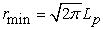(4.1)

the maximum momentum should be the result of (4.1).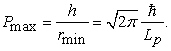(4.2)

Planck gravitation theory just can solve the problem of ultraviolet divergence in quantum field theory. This is a very shocking result.

### 5. Gravitation Quantization

Planck gravitation theory is already a quantum theory. As long as it is a quantum particle, it produces gravitation. And, in 4-dimensional space, each particle produces the same gravitation, regardless of particle type, regardless of particle energy, regardless of particle mass. The strength of gravitation is determined entirely by the three constants of theG and C. Planck gravitation has Lorentz invariance. Does gravitation need to be quantized? Even if gravitation need to be quantized, it should be a form of second quantization.

Planck gravitation actually has a property similar to space quantization. Each particle has an intrinsic property of Planck Length. For other particles, the minimum distance can only be close to the distance toThe distance can no longer be less thanEach particle represents a space hole. The radius of the hole isThis view is actually similar to space quantization. The space seems to be separated into one empty unit. Planck Length is the radius of the hole unit. But, obviously, this view is just a visual understanding, not a physical nature. The physical origin of this view is not because space is really quantum, but actually because of the special nature of Planck gravitation. Space is not actually quantum. The quantization is Planck gravitation. If there are no particles in a space region, the region of this space does not have a gravitational hole, and the quantization of space is not shown. Quantum is not the space, but it is the particles. This is entirely in line with quantum mechanics. In contrast, space-time quantization does not align with quantum mechanics. From Planck gravitation theory, space-time quantization is a wrong method of gravitation quantization.

Planck gravitation can also be understood as a special symmetry breaking effect. Because particles have an intrinsic property of hole, the radius of the hole is Planck Length. Therefore, the space symmetry is broken, thus causing the symmetry breaking of the particle's wave function. This breaking effect manifests itself in gravitation. This can be seen from (2.5). This symmetry breaking can be understood as a kind of Lorentz symmetry breaking. Does this symmetry breaking effect need to be second quantized? Is there a gravition? There are doubts. From Planck gravitation, there is no gravition. There is a big difference between Planck gravitation and electromagnetic forces. Planck gravitation is a special symmetry-breaking effect. There is no particle for exchanging gravitation. So, there is no gravition. Gravitation is a special symmetry breaking effect of the particle's wave function. This view can only be understood in quantum mechanics. We can understand gravitation in this way. Gravitation does not act on particles, but on the wave function of particles.

Of course, this is only the author's current thinking, but this is not the final conclusion. Whether gravitation can yet be second quantization, it requires further study.

### 6. Verification of Planck Gravitation Theory

Planck gravitation theory is a special theory of gravitation. Can it have a different result than other theory of gravitation? How to conduct an experiment to test this theory? In particular, how can the difference between Planck gravitation and General Relativity be distinguished from experiments?

Microscopically, Planck gravitation is very weak. The gravitational strength is only great when it is close to Planck Length. However, current human experimental technology can’t reach the Planck Length. Therefore, this type of experimental validation cannot be performed.

On the macro level, projection gravitation has a different derivation result from General Relativity. This result has been calculated in detail in the author's projection gravitation paper 1. See the author's paper for a detailed derivation calculation process. Projection gravitation is a derivative result of Planck gravitation. Therefore, the result of projection gravitation can also be used to verify Planck gravitation theory. Projection gravitation predicts that the gravitation of a planet is associated with the eccentricity of the orbit. The greater the orbital eccentricity, the greater the gravitation the planet receives. This result is different from General Relativity. This result can be used to make experimental measurements and test the validity of Planck gravitation theory.

In General Relativity, the planet moves around the star, taking a stage approximation, and the resulting planetary orbit equation is (6.1).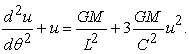(6.1)

Here, u=1/r, L is the orbital angular momentum of the planet, and M is the mass of the star.

However, in the projection gravitation, the calculated planetary orbital equation is (6.2).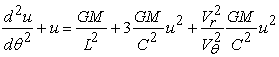(6.2)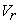is the radial velocity of planetary motion, and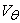is the lateral velocity of planetary motion. The ratio of these two speeds is determined by orbital eccentricity. There is one more item to projection gravitation. This can be used to distinguish between projection gravitation and General Relativity. Selecting planets with a large eccentricity, measuring the orbit of the planet, and compare with (6.1), and compare with (6.2), can be distinguished, whether general relativity is more in line with the measurement results, or projection gravity is more in line with the measurement results. For a detailed derivation of (6.2), read the projection gravitation paper published earlier by the author 1.

### 7. Black Hole Entropy

In the projection gravitation theory, the author has proposed a very simple black hole model 2, 3, which can be easily derived out the black hole entropy formula. A black hole is actually an empty hole. Outside particles are infinitely close to the hole, but never enter the inside of the hole. This is the concept of black holes in projection gravitation, which is completely different from the concept of black holes in General Relativity. There is a big difference between the two.

In the projection gravitation theory, a collection of particles that meet a special merger condition can form a special thermodynamic particle system. The entropy of this special thermodynamic system is the black hole entropy. This special merger condition is (7.1).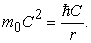(7.1)

Here,is the rest mass of particles merging into a black hole, r is the radius of black hole.

From this black hole model, the entropy formula (7.2) is obtained by calculation. This formula, compared with the Hawking entropy formula, is only one constant difference. For a detailed description and calculation of this black hole model, read the author's previous paper 2 3 on black hole entropy.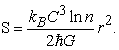(7.2)

Here, r is the radius of black hole, n is the degree of freedom of the particles formed black hole.

Moreover, in the projection gravitation, from the merging model formed black hole, without the need for other man-made assumptions, we can simply derive a formula (7.3). In (7.3), S is the entropy of black hole,is the rest mass of particles merging into a black hole, r is the radius of black hole. This formula (7.3), compared with the Verlinde’s entropy gravity proposal formula, is only one constant difference. It is proved that the entropy gravity proposal formula is only a derivation of the black hole entropy. Entropy gravity theory is not an independent gravity theory.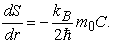(7.3)

Projection gravitation is a derivative result of Planck gravitation. Therefore, the results of black hole entropy obtained in projection gravitation, is the result of Planck gravitation also. Therefore, Planck gravitation can simply derive out the black hole entropy formula. The black hole entropy model in projection gravitation is fully in line with quantum mechanics, and is fully in line with statistical mechanics, and there is no problem of information loss. Therefore, the black hole model in projection gravitation is more reasonable and logically consistent than the black hole model in General Relativity.

For a detailed description of the black hole entropy in projection gravitation, please read the author's previous paper 2, 3.

The black hole in projection gravitation is in 3-dimensional space. Is there a black hole in 4-dimensional space? If so, what is the property of the black hole, and what is the property of the black hole entropy? This problem needs further study.

### 8. Projection Action

From Planck gravitation formula (2.11) to derive the projection gravitation formula (2.7), a projection process is required to export this result. This projection process is a projection action from 4-dimensional space to 3-dimensional space. The space currently observed by humans is actually a projection of 4-dimensional space. Or, the 3-dimensional space currently observed by humans is a flat membrane of 4-dimensional space.

In 4-dimensional space, there are two different types of particles.

The first type particle, the particle has no momentum component in the direction of the 4th dimension, only has the momentum on the flat membrane. Or, the direction of the particle's motion is entirely on the flat membrane, or parallel to the flat membrane. The projection action does not change the property of the particle. This particle is a particle with zero rest mass observed in 3-dimensional space. For example, photons.

Another type particle, the particle has momentum component in the direction of the 4th dimension. The momentum component in the direction of the 4th dimension is projected into 3-dimensional space, and as observed in 3-dimensional space, the momentum component remains the same. In 3-dimensional space, this momentum component is characterized by the property of rest mass. As seen in 3-dimensional space, particles can only change the momentum on 3 dimensions, the momentum component on 4th dimension does not be changed. The Compton wavelength of the particle in 3-dimensional space is actually the de Broglie wavelength of the particle in the direction of the 4th dimension of spaceM is rest mass of particles. As you observe in 4-dimensional space, there are no static particles at all. It is similar to the absence of static photons in 3-dimensional space. Particles that are static in 3-dimensional space are still moving in 4D space.

It is because of this projection action, the gravitation observed by humans in 3-dimensional space is distorted and altered. In 4-dimensional space, particles do not have a rest mass, and the resulting gravitation has nothing to do with the rest mass of particles. But the gravitation force observed in 3-dimensional space is associated with the rest mass of particles. The rest mass is the result of this projection action. This projection action not only results in a rest mass, but also causes gravitation to be related to the rest mass. But, in fact, the correlation between gravitation and rest mass is really just an illusion, not the original nature of gravitation. The gravitation observed in 3-dimensional space is a distorted, not a real. The truth of gravitation can only be understood in 4-dimensional space.

The transition from (2.11) to (2.7) is actually the transition from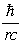to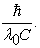The result of this transition is the emergence of rest mass M. The projection action causes particles to lose degrees of freedom on the 4th space dimension. This disappear degrees of freedom translate into the effect of rest mass in 3-dimensional space.

Another consequence of this transition is that the quantum properties of gravitation are obscured and hidden. This led to the belief that gravitation was a classical force, leading to the belief that gravitation had nothing to do with quantum mechanics. Humans believe that gravitation also needs to be quantized. But this is an illusion. But gravitation is already a quantum force in fact. A quantum particle produces a quantum force, which is gravitation.

On the other hand, for this projection action, after all, it is only a physical model. We can’t absoluteitize it. The role of this projection action is actually to provide a transition from (2.7) to (2.11). The essence of the projection action is to provide a transition between (2.11) and (2.7). However, it can’t be 100% sure that this action must be 4-dimensional space projection to 3-dimensional space. Perhaps in 3-dimensional space, there is an unknown physical process that can provide the transition between (2.11) and (2.7), with the same effect as the projection action. There is also a possibility, and it cannot be ruled out.

But, projection from 4-dimensional space into 3-dimensional space, such a perspective, is the easiest physical perspective to understand, and also the physical view to inspire author to understand the gravitation.

What physical process exists behind the projection action? What is the physical essence of projection action? It is an issue that requires further study.

### 9. Conclusion

The author has previously published a projection gravitation theory. Projection gravitation theory, though, can derive many meaningful results. But projection gravitation theory doesn't answer all the questions of gravitation. To the property of the gravitation produced by photons, projection gravitation theory can’t answer. Projection gravitation theory is not yet a complete gravitation theory.

Starting from projection gravitation theory, the author puts forward a more universal gravitation theory, which is Planck gravitation theory. The projection gravitation theory is only a derivation of Planck gravitation theory. Planck gravitation is the truth of gravity. Planck gravitation reveals that the origin of gravitation is actually the quantization of particles. Gravitation is already a quantum force.

Based on Planck gravitation theory, the author deduces the gravitation formula produced by photons. Planck gravitation theory has a special property similar to the quantization of space. Planck gravitation theory can give a maximum momentum, can solve the problem of ultraviolet divergence in quantum field theory from the principle without the need for artificial renormalization. Planck gravitation theory can derive the black hole entropy formula. The authors expect more researchers to join the study of Planck gravitation theory, revealing more of the magical property of gravitation.

### References

  Xiao Lin Li. Projection Gravitation, a Projection Force from 5dimensional Space-time into 4-dimensional Space-time International Journal of Physics, 2017, 5(5), 181-196. In article  Xiao Lin Li. Empty Hole Entropy and Black Hole Entropy in Gravitation International Journal of Physics, 2018, 6(5), 155-160. In article View Article  Xiao Lin Li. Black Hole Entropy, Verlinde’s Entropy Gravity Proposal and Unruh Formula in Projection Gravitation International Journal of Physics. 2019, 7(2), 58-62. In article View Article  M. Planck. The Theory of Radiation. Dover 1959 translation, 1906. In article  Bekenstein, Jacob D. (April 1973). Black holes and entropy Physical Review D 7(8): 2333-2346. In article View Article  Bardeen, J. M.; Carter, B.; Hawking, S. W. The four laws of black hole mechanics Comm. Math. Phys. 31 (1973), no. 2, 161-170. In article View Article  E. P. Verlinde. On the Origin of Gravity and the Laws of Newton JHEP 1104 (2011) 029 [arXiv:1001.0785 [hep-th]]. In article View Article  David Chandler introduction to modern statistical mechanics ISBN-13: 978-0195042771. In article  R. P. Feynman,R. B. Leighton, M. Sands The Feynman Lectures on Physics (Volume I, II,III) ISBN 9787506272476, ISBN 9787506272483, ISBN 9787506272490. In article  Steven Weinberg. The Quantum Theory of Fields ISBN 0-52155001-7. In article  Steven Weinberg. GRAVITATION AND COSMOLOGY: PRINCIPLES AND APPLICATIONS OF THE GENERGAL THEORY OF RELATIVITY ISBN 0-471-92567-5. In articleThis work is licensed under a Creative Commons Attribution 4.0 International License. To view a copy of this license, visit http://creativecommons.org/licenses/by/4.0/